# Isotopy (in topology)

This term may also have other meanings, see Isotopy.

A homotopy of a topological spacewith respect to a topological spaceis a continuous family ( indexed by \$t\$,, here and throughout )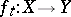of homeomorphisms fromonto subsets of. Analogously, an isotopy is a fibrewise-continuous mapping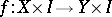such thattakes the fibre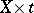homeomorphically onto a subset of the fibre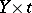. An isotopyfor which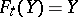for eachis called an isotopy of the space.

By a covering (or enveloping) isotopy for an isotopyone understands an isotopy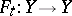such that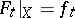. Two imbeddingsofintoare said to be isotopic if there exist a covering isotopyfor which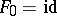,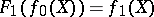. Two spaces,are said to be isotopy equivalent if there exist imbeddingsandsuch that the composites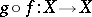and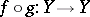are isotopic to the identity mapping. Isotopy-equivalent spaces are also called spaces of the same isotopy type (by analogy with the homotopy type). If two spaces are homeomorphic, then they are isotopy equivalent; however, there are non-homeomorphic spaces of the same isotopy type, for example, an-dimensional ball and the same ball with a line segment glued to it (at its ends). Any homotopy invariant is an isotopy invariant, but there exist isotopy invariants, for example dimension, that are not homotopy invariants.

The fundamental problem in isotopy theory is the isotopy extension problem, that is, the problem of the existence of an isotopy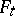covering a given isotopy. This problem, like the general problem of finding a complete system of isotopy invariants for imbeddings, is most often considered in the category of topological manifolds and its subcategories of piecewise-linear or differentiable manifolds.

A topological isotopy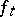of a manifold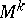with respect to a manifold,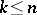, can be extended to a covering isotopy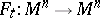if and only if the corresponding fibrewise imbedding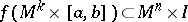is locally flat (cf. Locally flat imbedding); hereis a subinterval of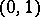. If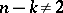and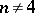, then the isotopyis covered by an isotopyprovided that the imbedding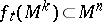is locally flat for any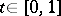. This is not true in codimension 2 (for example, "tying a knot" on a circle in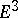), and therefore additional homotopy hypotheses are required for the existence of a covering isotopy. Sufficiently locally close flat imbeddings are isotopic for.

The existence theorem for a piecewise-linear isotopy is formulated in similar fashion in the general case (under the natural condition that the corresponding fibrewise imbeddings be locally flat in the piecewise-linear sense). If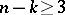, then a piecewise-linear isotopy can always be extended, since in these codimensions a piecewise-linear imbedding is locally flat in the piecewise-linear sense. Foror 1 it is necessary to additionally suppose that the imbeddingsbe locally flat in the piecewise-linear sense, since in these codimensions, a piecewise-linear imbedding need not to be locally flat even in the topological sense, for example, a cone over a knot.

A differentiable isotopy can always be extended to a differentiable covering isotopy.

The problem of finding a complete system of isotopy invariants for imbeddings of a manifoldinhas only been solved in a few particular cases. Thus, every locally flat (in the topological sense) imbedding of spheres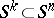foror for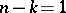,, is isotopic to the standard sphere, while if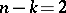,, then a locally flat sphere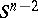in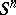is isotopic to the standard sphere if and only if the complement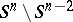has the homotopy type of the circle (the Stallings–Brown theorem). In codimension 2 there can exist non-isotopic knots. In precisely the same way the Zeeman–Stallings theorem on the piecewise-linear isotopy (unknottedness) of piecewise-linear spheres can be stated.

An invariant of piecewise-linear isotopy is finer than an invariant of topological isotopy. Thus, the problem of topological isotopy of an arbitrary homeomorphism ofto the identity has been solved in the positive sense for, and under the same restriction the isotopy has been proved of any two orientation-preserving homeomorphism of the sphereonto itself. In the piecewise-linear case, these statements can be proved by elementary methods without any restrictions. Sufficiently close homeomorphisms of a topological manifold onto itself are isotopic; on the other hand, there exist arbitrarily close, piecewise-linear non-isotopic, piecewise-linear homeomorphisms of piecewise-linear manifolds, for example, of the-dimensional tori for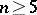.

In contrast with the topological and piecewise-linear cases, it is by no means true that any two diffeomorphisms of an-sphere onto itself are differentiably isotopic. The isotopy classes of differentiable imbeddings of a sphere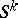intohave been studied in detail for any. If, then there exist a topological space,, such that the homotopy groups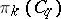are in one-to-one correspondence with the imbedding classes ofinto. Herefor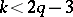, whileis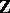or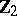depending onbeing even or odd. Thus, the standard-sphere, imbedded in, can forbe knotted in the differentiable sense, that is, there exist imbeddingsthat are not differentiably isotopic to the standard imbedding. These knots are called Haefliger knots. If, then the differentiable knots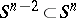can be knotted in the topological sense; however, there are considerably more of these than of the topological or piecewise-linear ones; for oddthey have been completely classified. If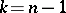,, then any differentiable imbedding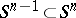is differentiably isotopic to the standard imbedding.

The fact that there exist diffeomorphisms ofonto itself that are not isotopic to the identity leads to the existence of non-trivial differentiable structures on spheres of dimension. Although every homeomorphism of,, onto itself can can be approximated by a diffeomorphism, not all close diffeomorphisms of a sphere are differentiably isotopic, that is, a diffeomorphism of a sphere onto itself can by an infinitesimal perturbation be transformed in one not isotopic to it.

How to Cite This Entry:
Isotopy (in topology). Encyclopedia of Mathematics. URL: http://encyclopediaofmath.org/index.php?title=Isotopy_(in_topology)&oldid=32175
This article was adapted from an original article by M.A. Shtan'ko (originator), which appeared in Encyclopedia of Mathematics - ISBN 1402006098. See original article